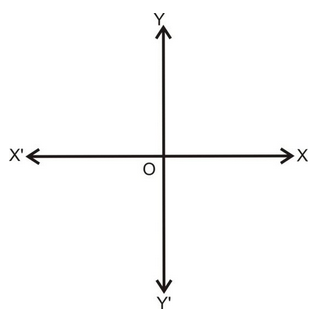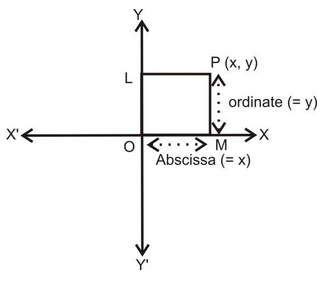# Rectangular Coordinates

## Coordinate Geometry of Class 10

Take two perpendicular lines X’ OX and Y’ OY intersecting at the point O. X’ OX and Y’ OY is called the co-ordinate axes. X’ O is called the X-axis, Y’ OY is called the Y-axis, and O is called the origin. Lines X’ OX and Y’ OY is sometimes also called rectangular axes.### CO-ORDINATES OF A POINT:

Let P be any point as shown in the figure. Draw PL and PM perpendiculars on Y-axis and X-axis, respectively. The length LP (or OM) is called the x - coordinate of the abscissa of point P and MP I is called the y - coordinate or the ordinate of point P. A point whose abscissa is x and ordinate is y is named as the point (x, y) or P(x, y).The two liens X’ OX and Y’ OY divide the plane into four parts called quadrants. XOY, YOX’ X’OY’ and Y’OX are, respectively, called the first, second, third and fourth quadrants. The following table shows the signs of the coordinates of pins situated in different quadrants :

 Quadrant X-coodrinate Y-coordinate Point First quadrant + + (+, +) Second quadrant - + (-, +) Third quadrant - - (-, -) Fourth quadrant + - (+, -)

### REMARKS:

1. The abscissa is the perpendicular distance of a point from the y-axis (i.e., positive to the right of the y-axis and negative to the left of y-axis)
2. The ordinate is the positive above the x-axis and the negative below the x-axis.
3. The abscissa of any point on the y-axis is zero.
4. The ordinate of any point of the x-axis is zero.
5. Co-ordinates of the origin are (0,0).

Do solve NCERT textbook for class 10 maths with the help of pw NCERT solutions for class 10 maths.